Chapter 3 Class 9 Coordinate Geometry

Class 9
Important Questions for Exam - Class 9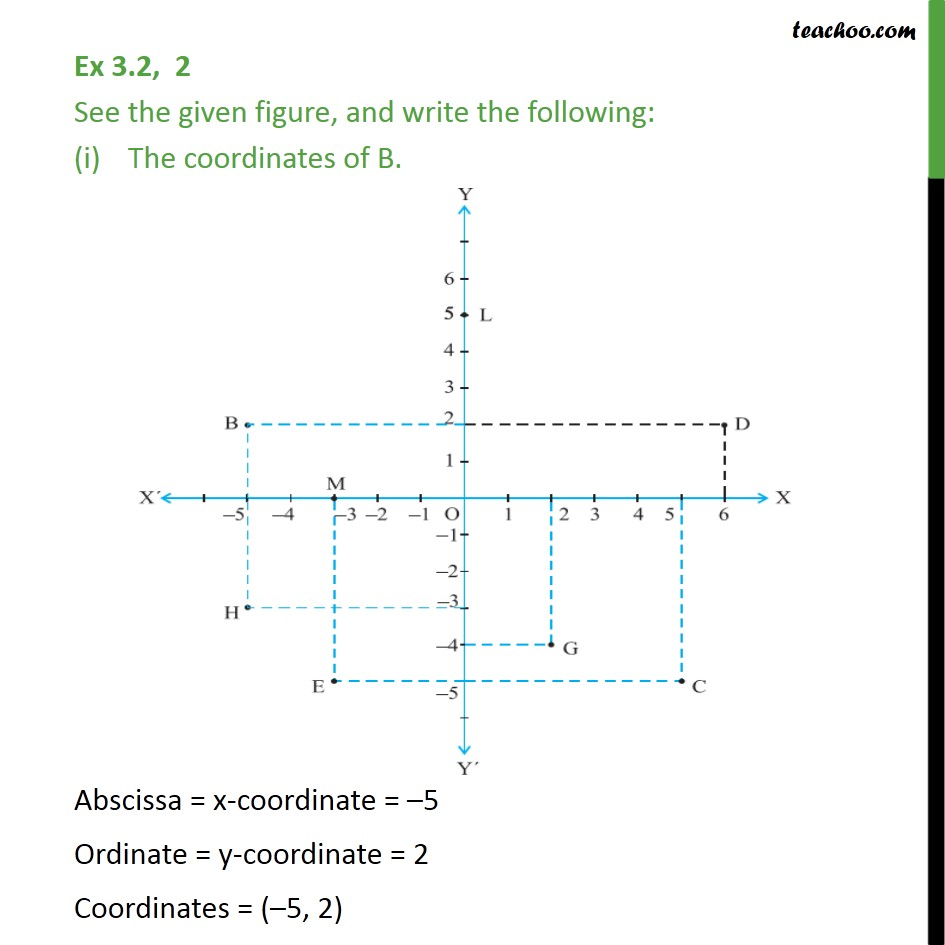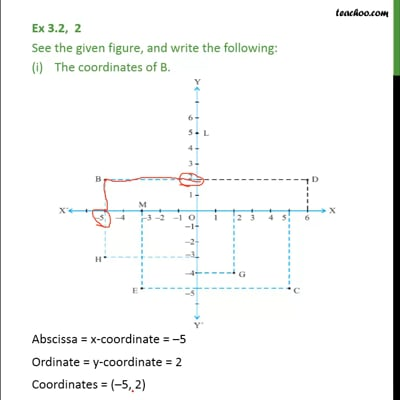This video is only available for Teachoo black users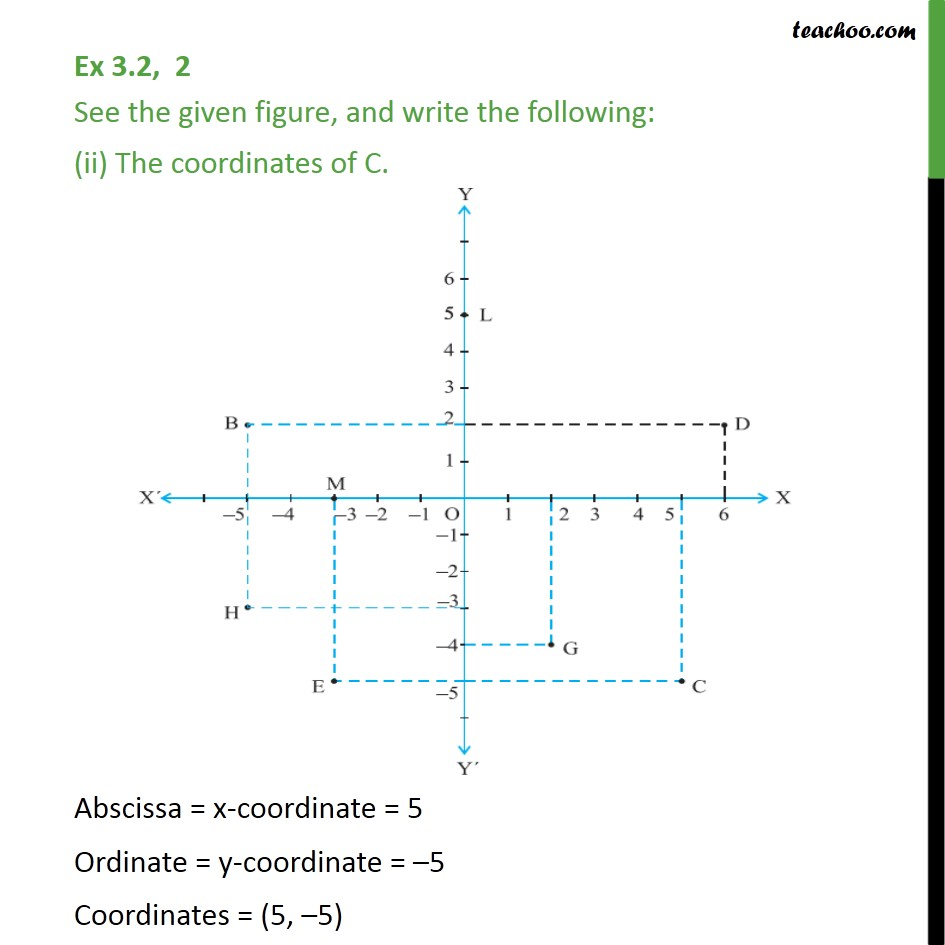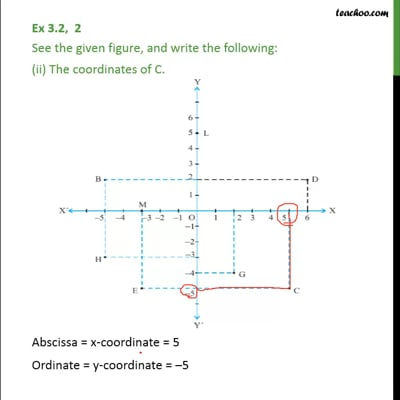This video is only available for Teachoo black users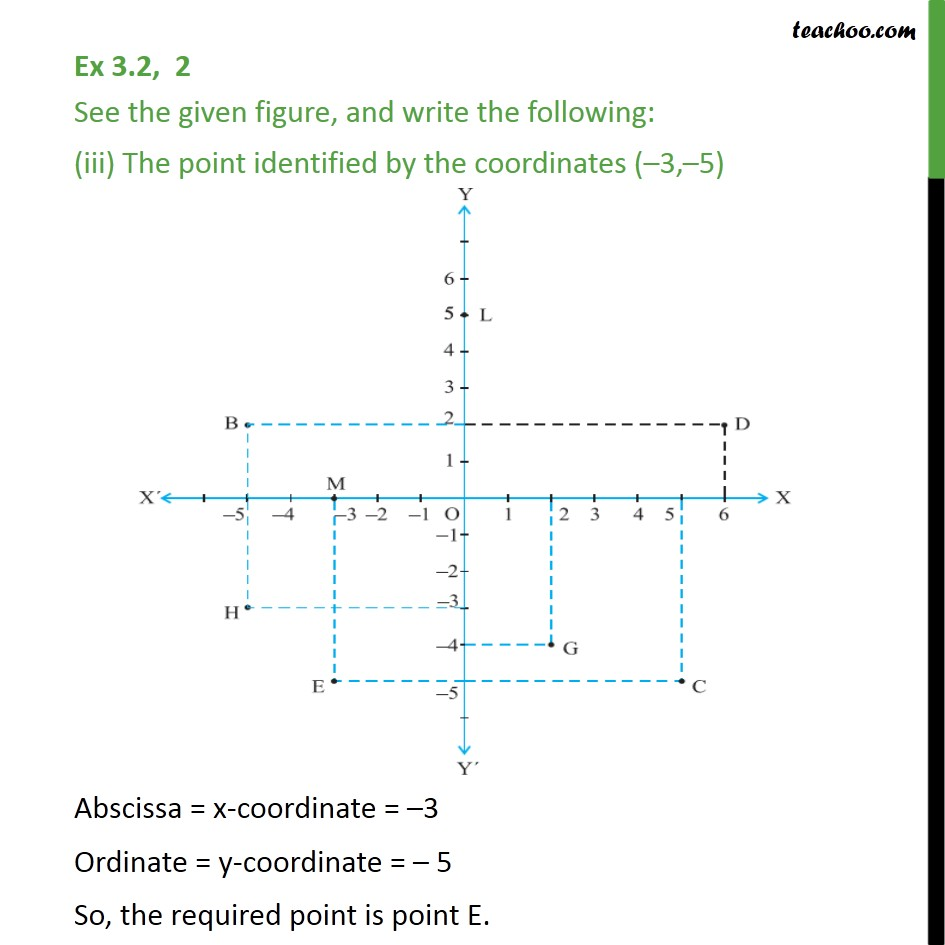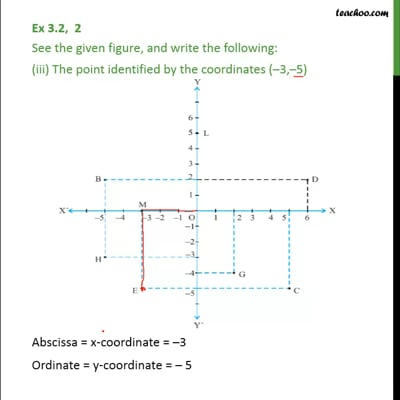This video is only available for Teachoo black users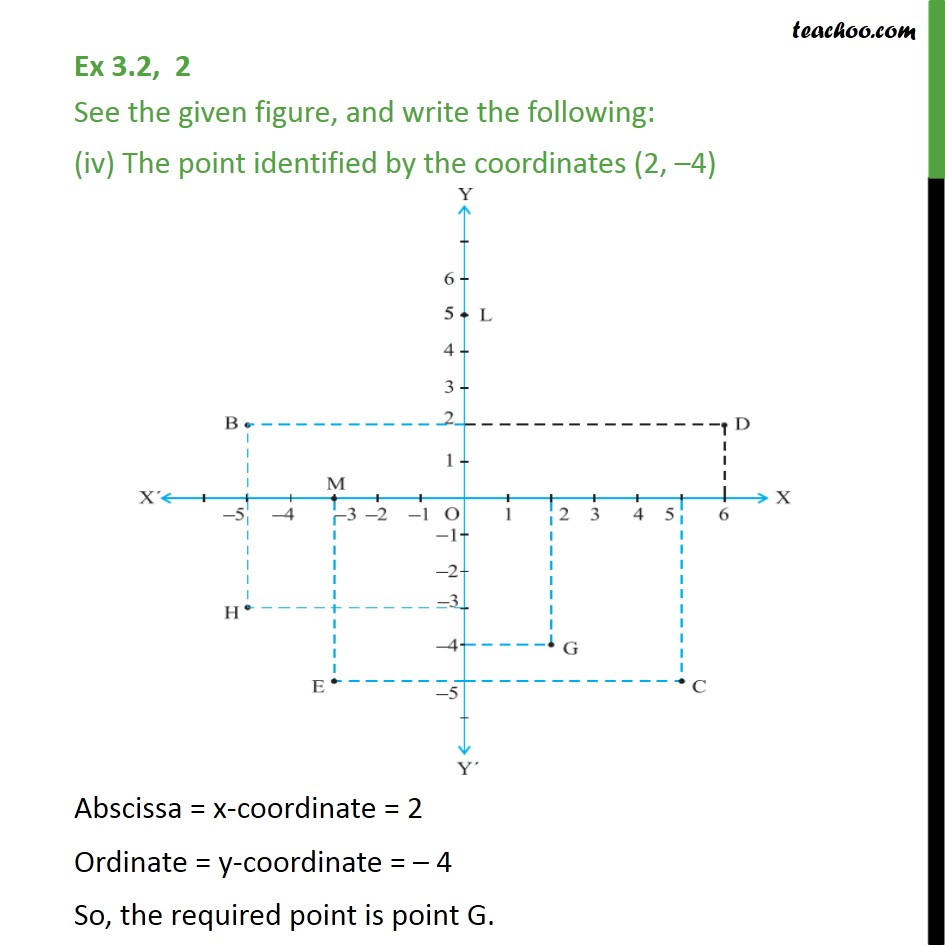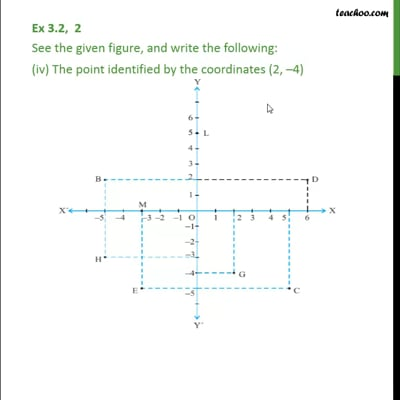This video is only available for Teachoo black users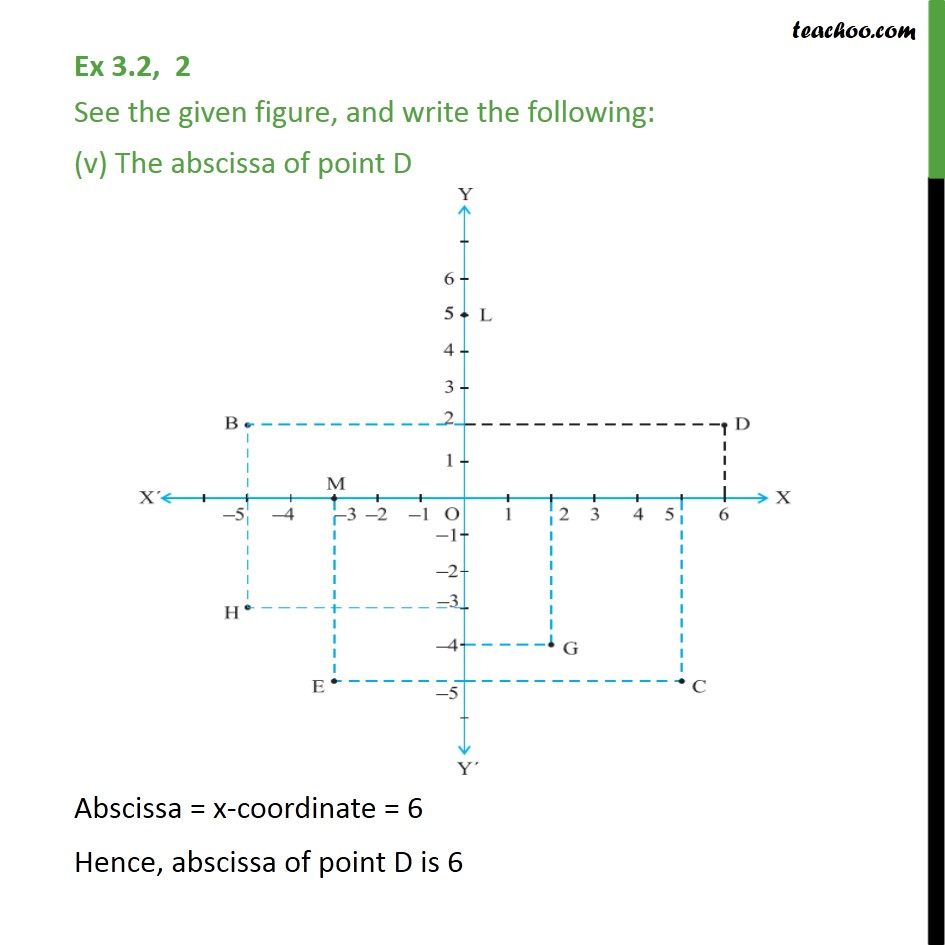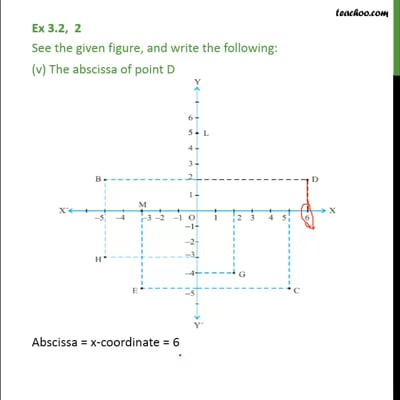This video is only available for Teachoo black users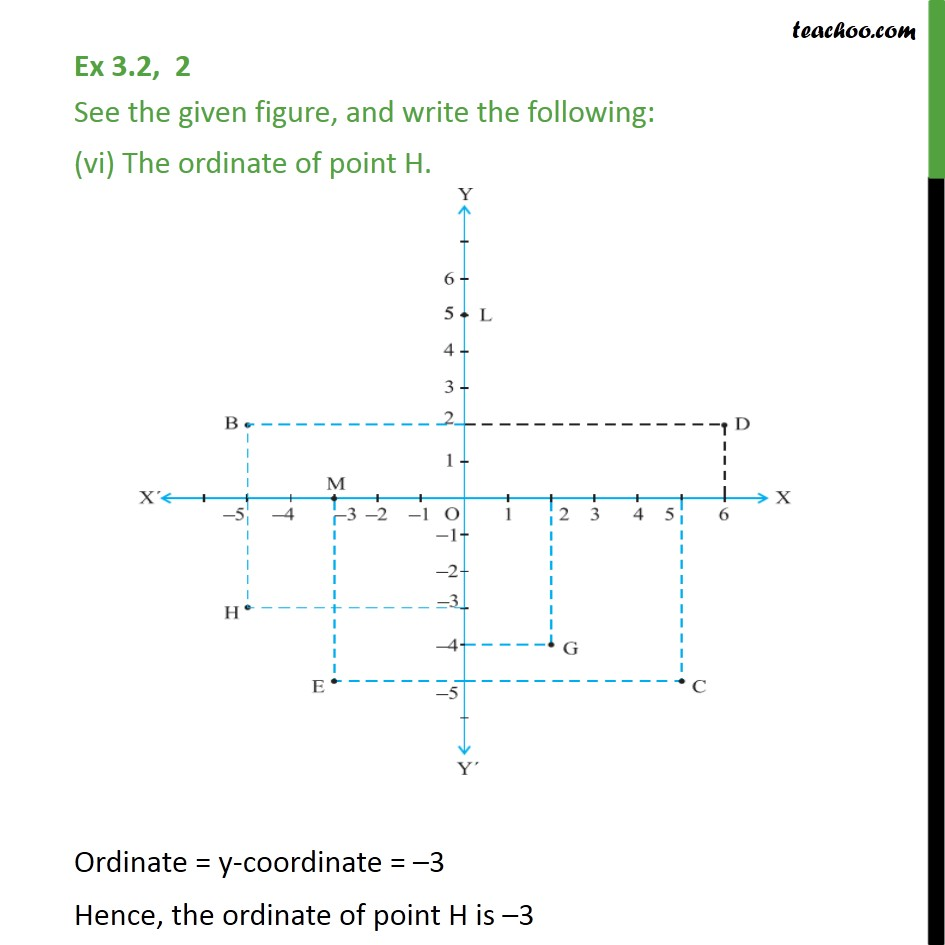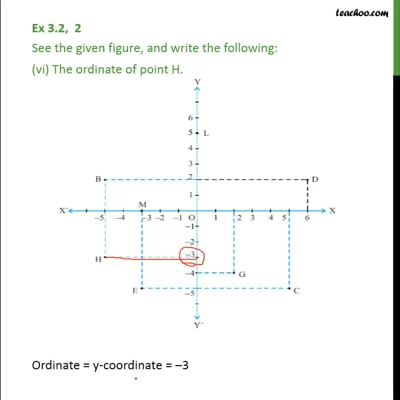This video is only available for Teachoo black users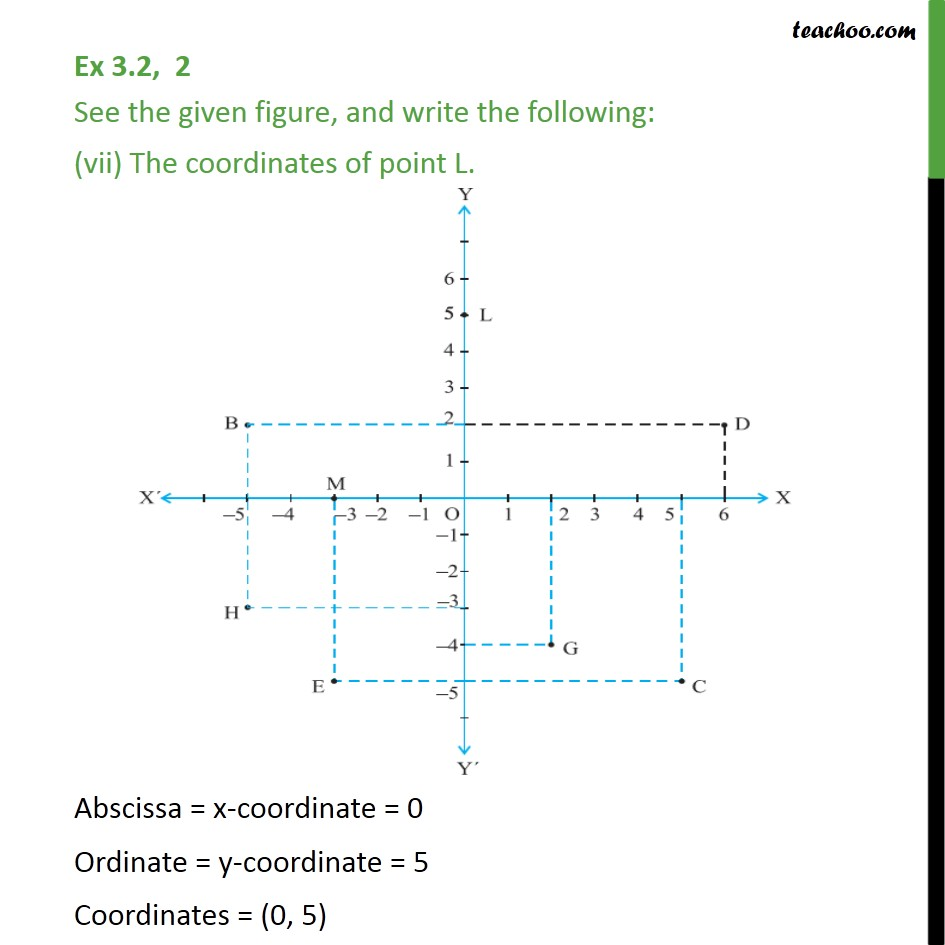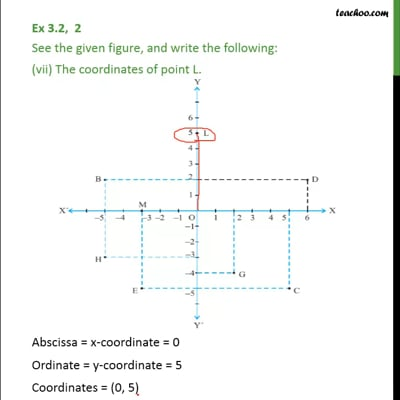This video is only available for Teachoo black users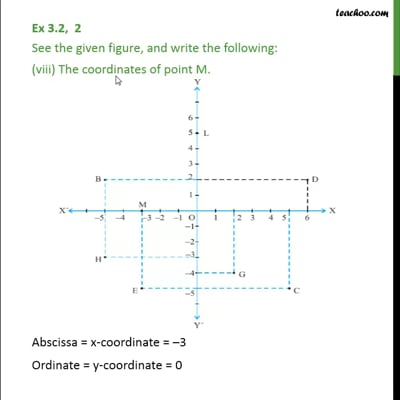This video is only available for Teachoo black users

Solve all your doubts with Teachoo Black (new monthly pack available now!)

### Transcript

Ex 3.2, 2 See the given figure, and write the following: The coordinates of B. Abscissa = x-coordinate = 5 Ordinate = y-coordinate = 2 Coordinates = ( 5, 2) Ex 3.2, 2 See the given figure, and write the following: (ii) The coordinates of C. Abscissa = x-coordinate = 5 Ordinate = y-coordinate = 5 Coordinates = (5, 5) Ex 3.2, 2 See the given figure, and write the following: (iii) The point identified by the coordinates ( 3, 5) Abscissa = x-coordinate = 3 Ordinate = y-coordinate = 5 So, the required point is point E. Ex 3.2, 2 See the given figure, and write the following: (iv) The point identified by the coordinates (2, 4) Abscissa = x-coordinate = 2 Ordinate = y-coordinate = 4 So, the required point is point G. Ex 3.2, 2 See the given figure, and write the following: (v) The abscissa of point D Abscissa = x-coordinate = 6 Hence, abscissa of point D is 6 Ex 3.2, 2 See the given figure, and write the following: (vi) The ordinate of point H. Ordinate = y-coordinate = 3 Hence, the ordinate of point H is 3 Ex 3.2, 2 See the given figure, and write the following: (vii) The coordinates of point L. Abscissa = x-coordinate = 0 Ordinate = y-coordinate = 5 Coordinates = (0, 5) Ex 3.2, 2 See the given figure, and write the following: (viii) The coordinates of point M. Abscissa = x-coordinate = 3 Ordinate = y-coordinate = 0 Coordinates = ( 3, 0)